21.* (1994 F 11) Branched hydrocarbons burn more smoothly than "straight chain" hydrocarbons in automotive internal combustion engines. Thus to make "high-test" gasoline 2,2,4-trimethylpentane (iso-octane) is added to n-octane [CH3(CH2) 6CH3]. Today, large amounts of the anti-knock compound iso-octane are produced from the reaction of isobutylene and isobutane: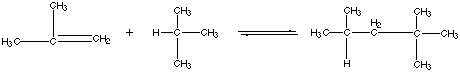At 25oC (298K) the gas-phase equilibrium constant is K = 4.3 x 10-6, and the heat of reaction (enthalpy of reaction) referenced to standard states is DHš = -78.6 kJ/mol.

A. Offer and explanation for the sign of DHš.

B. Calculate DGo at 25oC for this reaction.

C. Calculate D So at 25oC for this reaction.

D. Offer an interpretation for the sign of DSo.

E. Assume that DHo and DSo are independent of temperature. With this approximation estimate DGo and hence the equilibrium constant K at 700oC.

F. To what extent does the reation go to completion at 700oC compared to 25oC?

G. The first attempts at making iso-octane industrially from isobutane and isobutylene were not greatly successful, but it was found that a higher yield of iso-octane was obtained by heating the reaction mixture to 7000C rather than operating at room temperature. Offer an explanation for this finding.

H. A method of choice for making iso-octane from isobutylene and isobutane is to react the latter in the presence of an acidic catalyst such as concentrated sulfuric acid (H2SO4) or hydrofluoric acid (HF). This catalyzed process is typically carried out at 00C — 100C. Explain how the catalyst affects the value of the equilibrium constant for this reaction.

23.* (1994 3 4) When a trace of FeSO4 is added to an aqueous solution of H2O2, the solution foams; a gas evolves. When a trace of ZnSO4 is added to another aqueous solution of H2O2, no reaction occurs.

A. Write equations showing the reactions that occur when FeSO4 is present:

B. Show that the reaction has a negative free energy change.

C. Explain the role of FeSO4 and why ZnSO4 has no effect.

24.* (1994 3 6)

A. Show that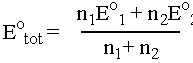B. Calculate the standard reduction potential for Fe3+ -->3e- --> Feo , given the following information.

 Half cell reaction Standard potential Fe(II) + 2e- --> Fe -0.41 Fe(III) + e- --> Fe(II) +0.77

25.* (1993 F Part I 8) Du Pont has developed a process for producing dilute aqueous hydrogen peroxide by hydrogenating oxygen over a palladium catalyst.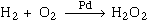A. Their engineers had to be careful about the materials in the reactor and product storage tank. The choices were stainless steel (containing Fe, Cr, and Mn) or glass. Which material did they choose? Explain using redox data. Your explanation should be quantitative.

Some redox potentials:

 Half reaction Standard reduction potential Fe3+ + e- --> Fe2+ 0.77V Cr3+ --> Cr2+ -0.50V Mn2+--> Mn -1.18V O2 + 2H+ + 2e- -->H2O2 0.69V

B. Demonstrate that the Du Pont process does not violate thermodynamics.

26.* (1993 F Part II 1) The hydrogen iodide molecule, HI, has a bond energy of 71 kcal/mole, which is intermediate between that of H2 (104 kcal/mole) and I2 (36 kcal/mole). Consider the gas-phase equilibrium reaction: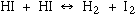A. Calculate the value of DH for this reaction in kcal/mole making use of the convention that positive DH means the absorption of heat by the system and negative DH means the liberation of heat by the system.

B. Present an argument why the value of DS for this reaction should be close to zero.

C. At room temperature (T = 300 K) HI gas 1 atm pressure in a quartz vessel is found to be stable with respect to disproportionation to H2 and I2, i.e., the concentration ratio [I2]/[HI] = [H2]/[HI] is less than 1%. Is this observation a consequence of thermodynamics or of slow kinetics? Discuss. Useful information: R = 1.987 cal šK-1 mole-1.

D. When HI gas in the quartz vessel is irradiated with ultraviolet light at a wavelength of 266 nm it decomposes into atoms: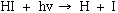where n is the frequency of the light and h = 6.626 x 10-27 erg sec is Planck's constant. Calculate in kcal/mole how much energy is released to the surroundings (negative DH) per mole of HI decomposed to H + I at 266 nm. Some useful information: E = hn, c = 3.00 x 1010 cm/sec, 1 erg/molecule = 1.439 x 1013 kcal/mole.

27.* (1993 3 1C)

C. At the time Stanford was founded, aluminum metal dinner plates were more expensive than solid silver plates whereas today the opposite is true. Explain.

28.* (1993 3 5)

A. What must the signs of DHo and DSo for a reaction at equilibrium that proceeds mostly to products for low T but not at high T (where T is the temperature)?

B. What must be the signs of DHo and DSo for a reaction at equilibrium that proceeds mostly to products for both low and high T?

29.* (1992 F 17) Consider the reaction diamond --> graphite. DH0 of diamond = 0.4532 kcal/mol and DH0 of graphite = 0 kcal/mol. DS0 of diamond = 0.6 cal/mol*K and DS0 of graphite = 1.37 cal/mol*K. Calculate the equilibrium constant for this reaction at 250C, 298K. As temperature increases, what happens to the equilibrium?

30.* (1992 3 1) The following polymerization reaction has DH° = -78.58 kJ/mol at room temperature. The equlilibrium constant is 4.3 x 10-6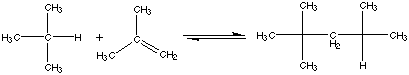A. Is this reaction exothermic or endothermic?

B. Calculate DG° at 25°C.

C. Calculate DS° at 25°C.

D. Calculate K at 700°C.

E. Offer an explanation for the different equilibrium constant values at the different temperatures.

31.* (1992 2 6)

A. Using the standard reduction potentials below, deduce the standard potential of the following reaction which occurs in aqueous solution: 2Fe3+ + 2I- --> Fe2+ + I2.

 I2 + 2e- --> 2I- 0.5355V 2Fe3+ + e- --> Fe2+ 0.771V

B. Using the standard reduction potentials below, deduce the standard potential of the reduction of Cr(III) to Cr metal.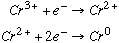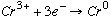E1=-0.424V E2=-0.90V E3=????V

32.* (1991 F 8) Why doesn’t molecular oxygen bond to metals in a 3+ oxidation state?

33.* (1991 F 13) The CO2 content of the atmosphere of Venus is about 75 atm. Some scientists theorize that this CO2 is produced via the decomposition of siderite and limestone on the planet.

A. Write an expression for the equilibrium constant for the decomposition of siderite (FeCO3).

B. Given the values of DH° and DS° in the table below, calculate the equilibrium constant for the decomposition of siderite at 500 K.

 Substance DH° J/mol DS° J/(K*mol) FeCO3 -7.39 x 105 92.8 FeO -2.658 x 105 57.3 CO2 -3.933 x 105 213.6

C. Write an expression for the equilibrium constant for the decomposition of limestone (CaCO3).

D. Given the values in the table below, compute the equilibrium constant for the decomposition of limestone at 500K.

 Substance DH° J/mol DS° J/(K*mol) CaCO3 -1.2059 x 106 92.8 CaO -6.349 x 105 39.7 CO2 -3.933 x 105 213.6

E. What do your calculations suggest is the partial pressure of CO2 on Venus? Is this in agreement with the measured pressure of 75 atm? Could the decomposition reactions account for the CO2 pressure on Venus? Discuss.

F. Are aqueous oceans expected to occur on Venus?

G. Sketch out a procedure for estimating the number of CO2 molecules in the Venusian atmosphere.

34.* (1991 3 2)

A. Use the data below to calculate the cell potential E0 for the reaction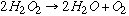.

 H2O2 + 2e- + 2H+ --> 2H2O E0 = 1.77V O2 + 2H+ + 2e- --> H2O2 E0 = 0.70V

B. What is the equilibrium constant for this reaction?

C. Contrary to intuition, dilute solutions of H2O2 are often stable. Offer an explanation for this.

35.* (1991 3 6)

A. Find the equilibrium constant K for the biologically important hydrolysis reaction:

ATP --> ADP + phosphate, which has

DG°=6.9 kcal/mol.

B. Calculate the free energy change for this reaction when [ADP] = 0.5 x 10-3, [phosphate] = 1.0 x 10-3, and [ATP] = 5 x 10-3. These are the concentrations that occur in a typical muscle cell. A catalyst for the hydrolysis reaction is present in the muscle cells.

C. Does the hydrolysis reaction of ATP to ADP reach equilbrium in a muscle cell? How efficient is the catalyst?

36.* (1990 F 2)

A. Illustrate with a balanced equation a reaction with negative enthalpy change and a positive entropy change:

C. Use equations to illustrate a reaction which can be carried out directly or electrochemically:

37.* (1990 F 7)

How can we make a reaction run "uphill"? Illustrate by giving an example of an industrial process which exploits this principle.

38.* (1990 F 13) Consider the reaction CaCO3(s) <–––> CaO(s) + CO2(g) at 1 atm CO2 and 298K. DH° for this reaction is +42.55 kcal/mole, DS° is +38.4 kcal/(mole*K).

A. Write an expression for the equilibrium constant for the above stated reaction in terms of DH° and DS°.

B. Calculate the sign and magnitude of DG°.

C. Offer an explanation for why DH° is positive for this reaction.

D. Offer an explanation for why DS° is positive for this reaction.

E. Would this reaction or its reverse proceed thermodynamically at 298°K?

F. Would an increase in temperature be expected to promote, retard, or have no effect on the reaction?

G. Would the addition of a catalyst be expected to increase, decrease, or leave unchanged the value of the equilibrium constant?

H. Would the addition of CaCO3 to the reaction mixture be expected to increase, decrease, or leave unchanged the value of the equilibrium constant?